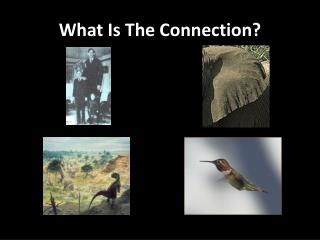# What Is The Connection? - PowerPoint PPT PresentationDownload PresentationWhat Is The Connection?

What Is The Connection?
Download Presentation## What Is The Connection?

- - - - - - - - - - - - - - - - - - - - - - - - - - - E N D - - - - - - - - - - - - - - - - - - - - - - - - - - -
##### Presentation Transcript

1. What Is The Connection?

2. A conceptual problem… • Q = How do cells feed and excrete? • A = Via their cell membranes • So therefore, shouldn’t cells require MORE surface area?

3. Lab: Assessing the Rate of Cellular Diffusion v. Differing Surface Area to Volume Ratios • Purpose: To determine how the size of a cell is related to its ability to perform diffusion • Method: Measurement of conductivity of the solution. The greater the rate of diffusion, the greater the rate of change in conductivity (as measured in µS/cm/sec)

4. Calculating S.A.: Vol Ratios • From block of sodium-infused agar, cut 3 2x2x2 blocks • Cut 2nd block into eight cubes • Cut 3rd block into 64 cubes • Calculate cumulative S.A.: Vol. ratios for each set of cubes

5. Collecting the data • Place the first 2cm3 cube into 300 mL of tap water • Immerse the conductivity probe into the tap water bath • Collect data, while STIRRING CONSTANTLY for 300 seconds • Determine the slope in µS/cm/sec

6. Processing/Interpreting The Data • Graph the rate (y-axis) vs. ratio of S.A.: Vol. (x-axis) • Answer the analysis questions in your lab notebook